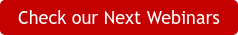Generator Expressions

Due to the success of list comprehensions, Python sought to create a mix between list comprehensions and generators. PEP 0289 introduces that idea, called the generator expression. In many of the use cases, we don’t need to have a full list created in memory, but to iterate over elements one at a time. List comprehensions return a list which is iterable, and can be iterated over many times. Generator expressions can only be iterated over once.

The construction of a generator expression is similar to a list comprehension, the only difference is that generator expressions use ( ) more parentheses than the use of [ ] brackets. Here is an example of a generator expression:In the example above, if I just print sum_num, it will just give me the generator object location in memory. So, I used the next method to iterate a through sum_num one at a time.

Generator Expression in a function
When a generator expression is used as a single argument in a function, then the surrounding parentheses isn’t needed. Like the example below:In the example above I used a generator expression as an argument in a sum function. Now, let’s try to put the second argument (10) in the sum function example:Oh my, I get an error. Basically, the error is saying that if you have multiple arguments in a function then the parentheses need to be included:No Variable Leaking
In Python 2, generator expressions have no variable leaking, unlike list comprehensions. Here is the same example from part one of the series but in a generator expression:Generator and Generator Expression

So, what is the difference between a generator and generator expression? Not much.

A generator can do what generator expression can, and in vice-versa. But some prefer to use a generator because it’s harder to step into a generator expression when debugging.

And here is the same example in a generator expression:List comprehensions are great for small to mid-size data sets and perform equal to generators. Now, if your data sets are large and increase due to adding new data, I recommend using a generator expression. Generator expressions tend to perform better as data volume increases because they don’t exhaust cache memory and they allow the re-use objects between iterations.

In the next part of Intermediate Python, we will take a glimpse into dictionary comprehensionsJaime Gabriel Jingco

Software Engineer/ Applied Labs Assistant Instructor### Min Yen